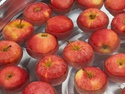## Samples and Probability

Type of Unit: Conceptual

# Prior Knowledge

Students should be able to:

• Understand the concept of a ratio.
• Write ratios as percents.
• Describe data using measures of center.
• Display and interpret data in dot plots, histograms, and box plots.

# Lesson Flow

Students begin to think about probability by considering the relative likelihood of familiar events on the continuum between impossible and certain. Students begin to formalize this understanding of probability. They are introduced to the concept of probability as a measure of likelihood, and how to calculate probability of equally likely events using a ratio. The terms (impossible, certain, etc.) are given numerical values. Next, students compare expected results to actual results by calculating the probability of an event and conducting an experiment. Students explore the probability of outcomes that are not equally likely. They collect data to estimate the experimental probabilities. They use ratio and proportion to predict results for a large number of trials. Students learn about compound events. They use tree diagrams, tables, and systematic lists as tools to find the sample space. They determine the theoretical probability of first independent, and then dependent events. In Lesson 10 students identify a question to investigate for a unit project and submit a proposal. They then complete a Self Check. In Lesson 11, students review the results of the Self Check, solve a related problem, and take a Quiz.

Students are introduced to the concept of sampling as a method of determining characteristics of a population. They consider how a sample can be random or biased, and think about methods for randomly sampling a population to ensure that it is representative. In Lesson 13, students collect and analyze data for their unit project. Students begin to apply their knowledge of statistics learned in sixth grade. They determine the typical class score from a sample of the population, and reason about the representativeness of the sample. Then, students begin to develop intuition about appropriate sample size by conducting an experiment. They compare different sample sizes, and decide whether increasing the sample size improves the results. In Lesson 16 and Lesson 17, students compare two data sets using any tools they wish. Students will be reminded of Mean Average Deviation (MAD), which will be a useful tool in this situation. Students complete another Self Check, review the results of their Self Check, and solve additional problems. The unit ends with three days for students to work on Gallery problems, possibly using one of the days to complete their project or get help on their project if needed, two days for students to present their unit projects to the class, and one day for the End of Unit Assessment.

Subject:
Mathematics, Statistics and Probability
Material Type:
Unit of Study
Level:
Middle School
7
Tags:
Causality, Independent and Dependent Events, Group Projects, 7th Grade Mathematics, Time, Estimation, Decimals, Fractions, Probability, Graphs, Assessment, Rain, Sampling, Mean Absolute Deviation, Theoretical Probability, Goals, Ratios, Measurement, Law of Large Numbers, Statistical Inference, Likelihood, Projects, Experimental Probability
Unit 6 Samples and Probability•••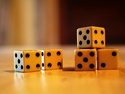••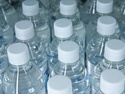•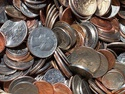••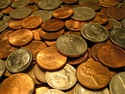••••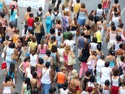•••••••••